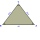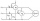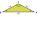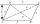# Cosine - high school - math problems

#### Number of problems found: 125

• Trapezoid 25Trapezoid PART with AR||PT has (angle P=x) and (angle A=2x) . In addition, PA = AR = RT = s. Find the length of the median of Trapezoid PART in terms of s.Calculate the content of a regular 15-sides polygon inscribed in a circle with radius r = 4. Express the result to two decimal places.
• The right triangleIn the right triangle ABC with right angle at C we know the side lengths AC = 9 cm and BC = 7 cm. Calculate the length of the remaining side of the triangle and the size of all angles.
• Isosceles triangleCalculate the size of the interior angles and the length of the base of the isosceles triangle if the length of the arm is 17 cm and the height to the base is 12 cm.
• Viewing angleThe observer sees a straight fence 60 m long at a viewing angle of 30°. It is 102 m away from one end of the enclosure. How far is the observer from the other end of the enclosure?
• The rescue helicopterThe rescue helicopter is above the landing site at a height of 180m. The site of the rescue operation can be seen from here at a depth angle of 52° 40 '. How far will the helicopter land from the rescue site?
• The angle of viewDetermine the angle of view at which the observer sees a rod 16 m long when it is 18 m from one end and 27 m from the other.
• Two grovesTwo groves A, B are separated by a forest, both are visible from the hunting grove C, which is connected to both by direct roads. What will be the length of the projected road from A to B, if AC = 5004 m, BC = 2600 m and angle ABC = 53° 45 ’?
• Decide 2Decide whether points A[-2, -5], B[4, 3] and C[16, -1] lie on the same line
• Angle of the body diagonalsUsing vector dot product calculate the angle of the body diagonals of the cube.
• What percentageWhat percentage of the Earth’s surface is seen by an astronaut from a height of h = 350 km. Take the Earth as a sphere with the radius R = 6370 km
• Power line poleFrom point A, the power line pole is seen at an angle of 18 degrees. From point B to which we get when going from point A 30m away from the column at an angle of 10 degrees. Find the height of the power pole.
• Tetrahedral pyramidDetermine the surface of a regular tetrahedral pyramid when its volume is V = 120 and the angle of the sidewall with the base plane is α = 42° 30'.
• A rhombusA rhombus has sides of length 10 cm, and the angle between two adjacent sides is 76 degrees. Find the length of the longer diagonal of the rhombus.
• Isosceles triangle 10In an isosceles triangle, the equal sides are 2/3 of the length of the base. Determine the measure of the base angles.Two wattmeters are connected to measuring power in a 3 phase balanced load. Determine the total power and power factor if the two wattmeters read 1000 watts each (1) both positive and (2) second reading is negative.
• Roof angleThe roof of the house has the shape of an isosceles triangle with arms 4 m long and the size of the base 6 m. How big an angle alpha does its roof make?
• Isosceles triangle 8If the rate of the sides an isosceles triangle is 7:6:7, find the base angle correct to the nearest degree.
• ParallelogramThe sides of the parallelogram are 8 cm and 6 cm long and the angle of the diagonals is 60°. What is its area?
• Children playgroundThe playground has a trapezoid shape, and the parallel sides have a length of 36 m and 21 m. The remaining two sides are 14 m long and 16 m long. Find the size of the inner trapezoid angles.

Do you have an interesting mathematical word problem that you can't solve? Submit a math problem, and we can try to solve it.

We will send a solution to your e-mail address. Solved examples are also published here. Please enter the e-mail correctly and check whether you don't have a full mailbox.

Please do not submit problems from current active competitions such as Mathematical Olympiad, correspondence seminars etc...

Cosine - math problems. Examples for secondary school students.